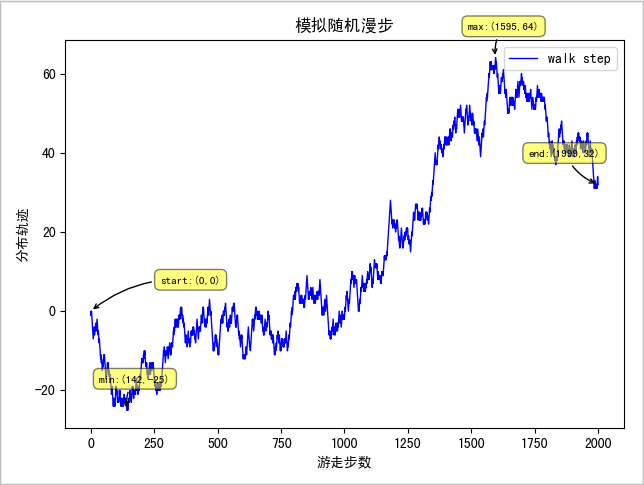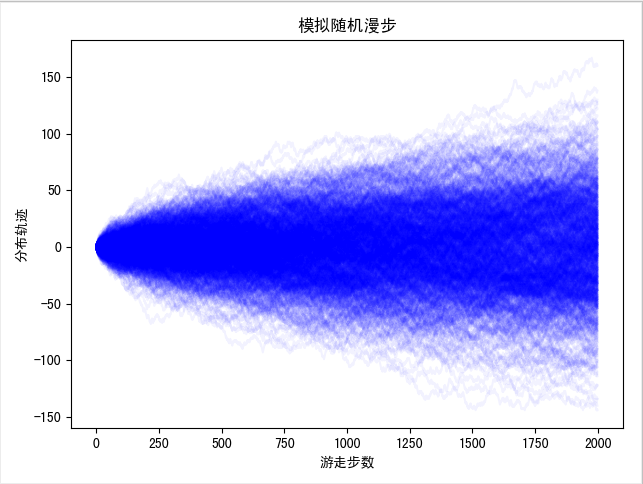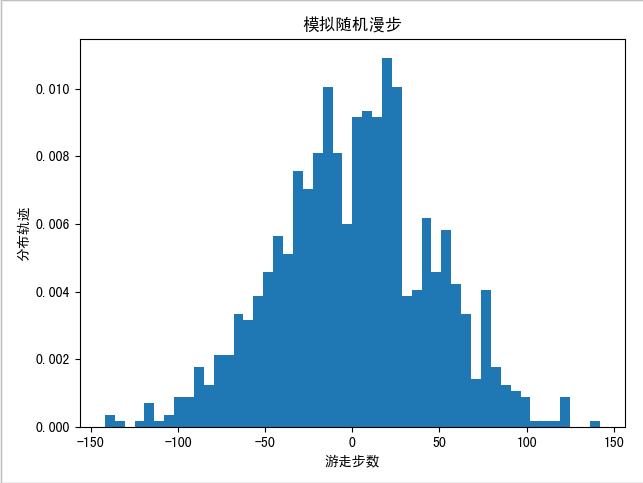股票价格在随机漫步吗？用 Python 来告诉你2. Python 随机数的生成

Python 内置的 random 模块和第三方库 NumPy 的 random 模块都提供了生成随机漫步序列的方法，分别为 random.randint() 和 numpy.random.randint() 函数。NumPy 中主要以 N 维数组对象 ndarray 存储数据的，ndarray 作为 NumPy 的核心，不仅具有矢量算术运算的能力，并且在处理多维的大规模数组时快速且节省空间。

 def list_test(): walk = [] for _ in range(1000000): walk.append(random.randint(0, 1)) def ndarray_test(): np.random.randint(0, 2, size=1000000) t1 = timeit('list_test()', 'from __main__ import list_test', number=1) t2 = timeit('ndarray_test()', 'from __main__ import ndarray_test', number=1) print("list：{}".format(t1)) # list：1.3908312620000003 print("ndarray：{}".format(t2)) # ndarray：0.009495778999999871

numpy.random.randint(low, high=None, size=None, dtype=’l’)

• 返回随机整数，范围区间为 [low,high]，包含 low，不包含 high
• 参数：low 为最小值，high 为最大值，size 为数组维度大小，dtype 为数据类型，默认的数据类型是 np.int
• high 没有填写时，默认生成随机数的范围是 [0，low]

 print("np.random.randint：\n {}".format(np.random.randint(1,size=5)))# 返回 [0,1) 之间的整数，所以只有 0 """ np.random.randint： [0 0 0 0 0] """ print("np.random.randint：\n {}".format(np.random.randint(1,5)))# 返回 1 个 [1,5) 时间的随机整数 """ np.random.randint： 2 """ print("np.random.randint：\n {}".format(np.random.randint(-5,5,size=(2,2)))) """ np.random.randint： [[-5 -3] [ 2 -3]] """

3. 预知随机漫步的规律

 draws = np.random.randint(0, 2, size=2000) print(f'random walk direction is {draws}') #random walk direction is [1 0 1 ... 0 1 0]def draw_random_walk(): walk_steps = 2000 walk_path = random_walk(walk_steps) # 统计漫步过程中，终点、前进和后退最大的距离 start_y = 0 start_x = 0 end_y = walk_path[-1] end_x = walk_steps-1 max_y = walk_path.max() max_x = walk_path.argmax() min_y = walk_path.min() min_x = walk_path.argmin() x = np.linspace(0, 2000, num=2000) # 绘制出漫步的足迹 plt.plot(x, walk_path, color='b', linewidth=1, label='walk step') # 添加标注 # 起点坐标 plt.annotate( 'start:({},{})'.format(start_x, start_y), xy = (start_x,start_y), xycoords='data', xytext=(+50, +20), textcoords='offset points', fontsize=8, bbox=dict(boxstyle='round,pad=0.5',fc ='yellow', alpha = 0.5), arrowprops=dict(arrowstyle='->', connectionstyle="arc3,rad=.2") ) # 终点坐标 plt.annotate( 'end:({},{})'.format(end_x, end_y), xy = (end_x,end_y), xycoords='data', xytext=(-50, +20), textcoords='offset points', fontsize=8, bbox=dict(boxstyle='round,pad=0.5', fc='yellow', alpha=0.5), arrowprops=dict(arrowstyle='->', connectionstyle="arc3,rad=.2") ) # 最大距离坐标 plt.annotate( 'max:({},{})'.format(max_x,max_y), xy = (max_x,max_y), xycoords = 'data', xytext = (-20, +20), textcoords='offset points', fontsize = 8, bbox=dict(boxstyle='round,pad=0.5', fc='yellow', alpha=0.5), arrowprops=dict(arrowstyle='->', connectionstyle="arc3,rad=.2") ) # 最小距离坐标 plt.annotate( 'min:({},{})'.format(min_x,min_y), xy = (min_x,min_y), xycoords = 'data', xytext = (-20, +20), textcoords='offset points', fontsize = 8, bbox=dict(boxstyle='round,pad=0.5', fc='yellow', alpha=0.5), arrowprops=dict(arrowstyle='->', connectionstyle="arc3,rad=.2") ) plt.legend(loc='best') plt.xlabel('游走步数') plt.ylabel('分布轨迹') plt.title(u" 模拟随机漫步 ") plt.show()4. 总结

作者介绍